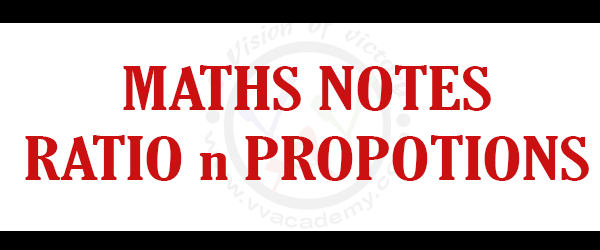## [:en]Concept Of Ratio And Proportion – MATHS NOTES [:]

[:en]

Concept Of Ratio And Proportion

# Concept Of Ratio And Proportion

What is Ratio?
A ratio is a relationship between two numbers by division of the same kind. The ration of a to b is written as a : b = a / b In ratio a : b , we can say that a as the first term or antecedent and b, the second term or consequent.
Example :  The ratio 4 : 9 we can represent as  4 / 9 after this 4 is a antecedent and , consequent = 9
Rule of ration :  In ratio multiplication or division of each and every term of a ratio by the same non- zero number does not affect the ratio.
Different type of ratio problem is given in Quantitative Aptitude which is a very essential topic in competitive exam. Under below given some more example for your better practice.
Anything we learn in our school days was basics and that is well enough for passing our school exams. Now the time has come to learn for our competitive exams. For this we need our basics but also we have to learn something new. That’s where shortcut tricks and formula are comes into action.What is Proportion?
The idea of proportions is that two ratio are equal.
If a : b = c : d, we write a : b : : c : d,
Ex. 3 / 15 = 1 / 5
a and d called extremes, where as b and c called mean terms.
Proportion of quantities
the four quantities a, b, c, d said proportion then we can express it
a : b = c : d
Then a : b : : c : d  <–> ( a x d ) = ( b x c )
product of means = product of extremes.
If there is given three quantities like a, b, c of same kind then then we can say it proportion of continued.
a : b = b : c the middle number b is called mean proportion. a and c are called extreme numbers.
So, b^2 = ac. ( middle number )^2 = ( First number x Last number ).

[:]